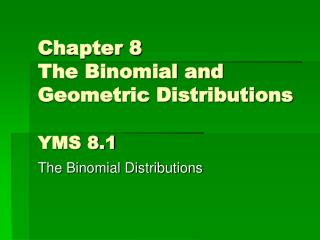Download PresentationChapter 8 The Binomial and Geometric Distributions YMS 8.1Chapter 8 The Binomial and Geometric Distributions YMS 8.1 - PowerPoint PPT Presentation

Download PresentationChapter 8 The Binomial and Geometric Distributions YMS 8.1
An Image/Link below is provided (as is) to download presentation

Download Policy: Content on the Website is provided to you AS IS for your information and personal use and may not be sold / licensed / shared on other websites without getting consent from its author. While downloading, if for some reason you are not able to download a presentation, the publisher may have deleted the file from their server.

- - - - - - - - - - - - - - - - - - - - - - - - - - - E N D - - - - - - - - - - - - - - - - - - - - - - - - - - -
Presentation Transcript

1. Chapter 8The Binomial and Geometric DistributionsYMS 8.1 The Binomial Distributions

2. Binomial Distribution • Distribution of the count X of successes in the binomial setting with parameters n and p • n is the number of observations and p is the probability of a success on any one observation • The possible values of X are the whole numbers from 0 to n • Denoted by B(n,p).

3. Binomial Setting • Each observation falls into one of just two categories, “success” or “failure” • There is a fixed number n of observations • The n observations are all independent • The probability of success, p, is the same for each observation

4. Binomial Calculations • pdf • probability distribution function which assigns value to single outcome X • cdf • cumulative distribution function which assigns value to range of X • Be careful of calculator entries when finding greater than or at least! p445 #8.4-8.8

5. Vocab and Simulations • Combination • order doesn’t matter • n choose k • Factorial • n! = n x (n-1) x (n-2) x 3 x 2 x 1 and 0! = 1 • Use randbin(1, p, n) to give 1 p% of the time and 0 (1-p)% of the time

6. Using Binomials • Binomial Probability • where the coefficient is a combination • Binomial Mean & Standard Deviation

7. Normal approximation to binomial distributions • As the number of trials n gets larger, the binomial distribution gets close to a normal distribution • Rule of thumb • N(np, ) can be used when n and p satisfy np≥ 10 and n(1 – p) ≥ 10 p454 #8.16-8.19 HW: 8.12-8.14, 8.26, 8.32-8.36

8. YMS 8.2 The Geometric Distribution

9. Geometric Setting • Each observation falls into one of just two categories: “success” or “failure” • The probability of success, p, is the same for each observation • The n observations are all independent • The variable of interest is the number of trials required to obtain the first success

10. Calculating geometric probabilities • probability that the first success occurs on the nth trial is • probability that it takes more than n trials to see the first success is

11. Geometric Mean & Standard Deviation 8.37 and 8.40 HW: p474 #8.44-8.46 Review: p479 #8.55-8.56, 8.60, 8.62-8.63